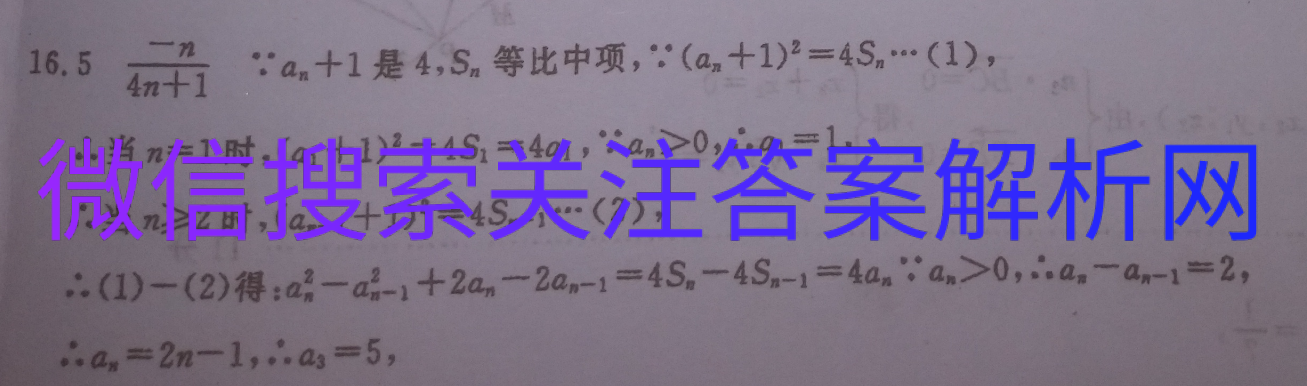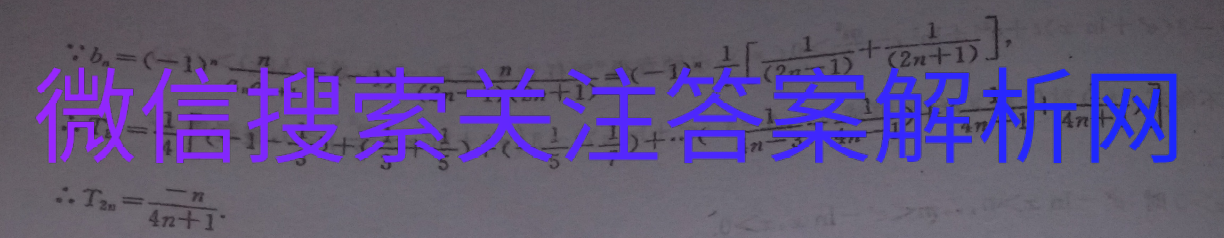# 衡水金卷2020-2021高三上学期六调

2021-03-07 16:4216.54n+1an+1是4,Sn等比中项,(an+1)2=4S…(1),当n=1时,(a1+1)2=4S1=4a1,∵an>0,…a1=1,∴当n≥2时,(an-1+1)2=45-1…(2),(1)-(2)得:a2-a2-1+2an-2an-1=4S-4Sn-1=4anan>0,,an-an-1=2,an=2n-1,∴a3=5∵bn=(-1)2=(-y)(2n-1)(2m+1)-1)2+anan+I(2∴.Tn=1)+(-11+an+)35)+(-1_1)+…()+(+4n-3、4n4n+,更多内容，请微信搜索关注。